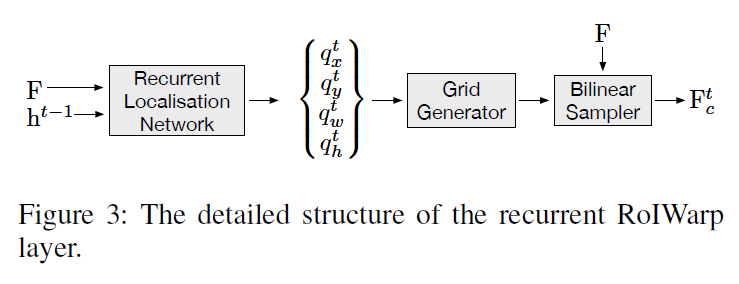# Contributions

• simple and efficient Char-Net for distorted scene text recognition. This network can be trained in end-to-end using only text images and their corresponding character labels.
• Hierarchical Attention Mechanism (HAM) that facilitates the rectification of individual characters and attends to the most relevant features for recognizing individual characters.
• A character-level encoder that **removes distortion of individual characters using a simple local spatial transformer.

# Overall Architecture

Char-net is consist of word-level encoder, character-level encoder and lstm-based decoder.# Word-Level Encoder (WLE)

• form of CNN
• encode entire text image

Authors wanted word-level encoder to make the feature map that has not only semantic information but also spatial information of each character. As the feature map of a deeper layer of CNN is more semantically strong and spatially coarse, WLE stacks convolutional feature map from different levels by several hyper-connections to increase spatial information.

# Character-Level Encoder (CLE)

• consist of local spatial transformer and CNN.
• The spatial transformer of the CLE make to handle complicated distortion of scene text.
• Previous work (global spatial transformer):
• rectify entire text image.
• used complicated thin-plate spline transformation to model different type of distortion.
• This paper (local spatial transformer):
• rectify distortion of an individual character.
• local STN can effectively model the complicated distortion of the scene text by simply predicting the rotation of each individual character

# Hierarchical Attention Mechanism (HAM)

HAM consists of the recurrent RoIWarp layer and character-level attention layer. Given the feature map $F$ of the text image produced by the word-level encoder, HAM aims at producing a context vector $z^t_c$ for predicting the label $y^t$ of the character being considered at time step $t$.

## Recurrent RoIWarp layer• This connects word-level encoder to character-level encoder. This aims at sequentially attending to a region of the feature map that corresponds to a character being considered at each time step.
• This works for extracting a small feature region that corresponds to the current character being recognized from the entire feature map produced by the word-level encoder and feeding it to the character-level encoder.

$\mathbf{z}_{c}^{t}=\sum_{i=1}^{W_{f}} \sum_{j=1}^{H_{f}} \alpha_{i j}^{t} \mathbf{F}_{i j}$ \begin{aligned} \mathbf{s}_{i j}^{t} &=\mathbf{w}^{\top} \operatorname{Tanh}\left(\mathbf{M h}^{t-1}+\mathbf{V F}_{i j}\right) \\ \boldsymbol{\alpha}^{t} &=\operatorname{SoftMax}\left(\mathbf{s}^{t}\right) \end{aligned}
• $z$: context vector
• $M, V$: parameter matrices
• $F$: feature map
• $s^t$: score map

### Reccurent Localisation Network (RLN)

RLN is responsible for recurrently locating each character RoI. RLN uses the score map $s^t$ to predict spatial information of each RoI.

$\left(q_{x}^{t}, q_{y}^{t}, q_{w}^{t}, q_{h}^{t}\right)=\operatorname{MLP}_{l}\left(\mathrm{s}^{t}\right)$
• $(q^t_x, q^t_y)$: the coordinates of the predicted centor of the character region
• $(q^t_w, q^t_h)$: the predicted width and height of the character region.
• MLP: Multi Layer Perceptron

As there is no direct supervision for RLN, it is hard to optimize RLN from scratch. Hence, the Authors pre-train a variant of the traditional attention mechanism to ease the difficulty in training the recurrent localization network. The generated weight set $\alpha^{t}$ can be interpreted as an attention distribution over all the feature points in the convolutional feature map.

Instead of generating an unconstrained distribution by normalizing the relevancy score map, we model the attention distribution as a 2-D Gaussian distribution and calculate its parameters by

$\left(\mu_{x}^{t}, \mu_{y}^{t}, \sigma_{x}^{t}, \sigma_{y}^{t}\right)=\operatorname{MLP}_{g}\left(\mathrm{s}^{t}\right)$

where $(\mu_x^t, \mu_y^t)$ and $(\sigma_x^t, \sigma_y^t)$ are center and standard deviations of distribution perspectively.

Similar to the traditional attention mechanism, this Gaussian attention mechanism can be easily optimized in an end-to-end manner. The authors then directly use the parameters of the Gaussian attention mechanism to initialize our RLN.

### Grid Generator and Bilinear Sampler

This target at cropping out the character of interest at each time step and warping it into a fixed size $W_c \times H_c \times C_f$.

## Character-Level Attention Layer (CLA)

• This connects the character-level encoder to LSTM-based decoder.
• This works for attending to the most relevant features of the feature map produced by character-level encoder and computing a context vector for the LSTM-based decoder.

CLA takes the responsibility of selecting the most relevant features from the rectified character feature map produced by the character-level encoder to generate the context vector $z_c^t$.

CLA is essential as it is difficult for the recurrent RoIWarp layer to precisely crop out a small feature region that contains features only from the corresponding character. Even though RLN predict warping region perfectly, the distortion exhibited in the scene text would cause the warped feature region to include also features from neighboring characters.

From experiment, the Authors find that features from neighboring characters and cluttered background would mislead the update of the parameters during the training procedure if they do not employ CLA, and this would prevent them from training Char-Net in an end-to-end manner.

# LSTM-based Decoder

• This recurrently predicts the ground truth labelling $y$ using LSTM layer.
$\mathbf{h}^{t}=\operatorname{LSTM}\left(\mathbf{h}^{t-1}, \mathbf{o}^{t-1}, \mathbf{z}_{c}^{t}\right) \qquad \qquad (1)$ $p\left(y^{t} | \mathbf{h}^{t-1}, \mathbf{o}^{t-1}, \mathbf{z}_{c}^{t}\right)=\operatorname{Soft} \operatorname{Max}\left(\mathbf{W}_{y} \mathbf{h}^{t}\right) \qquad \qquad (2)$
• $L$: set of labels
• $h^{t-1}$, $h^t$: previous, current hidden states
• $W_y$: parameter matrix
• $y^t$: label of the current predicted character
• $o^{t-1}$: one hot encoding of the previously predicted character. implicitly introduces a (learned) language model to assist the prediction of each character.
$p(\mathbf{y} | \mathbf{I})=\prod_{t=1}^{T+1} p\left(y^{t} | \mathbf{h}^{t-1}, \mathbf{o}^{t-1}, \mathbf{z}_{c}^{t}\right) \qquad \qquad (3)$

In the training process, we minimize the sum of the negative log-likelihood of Eq. (2) over the whole training dataset. During testing, we directly pick the label with the highest probability in Eq. (3) as the output in each decoding step.